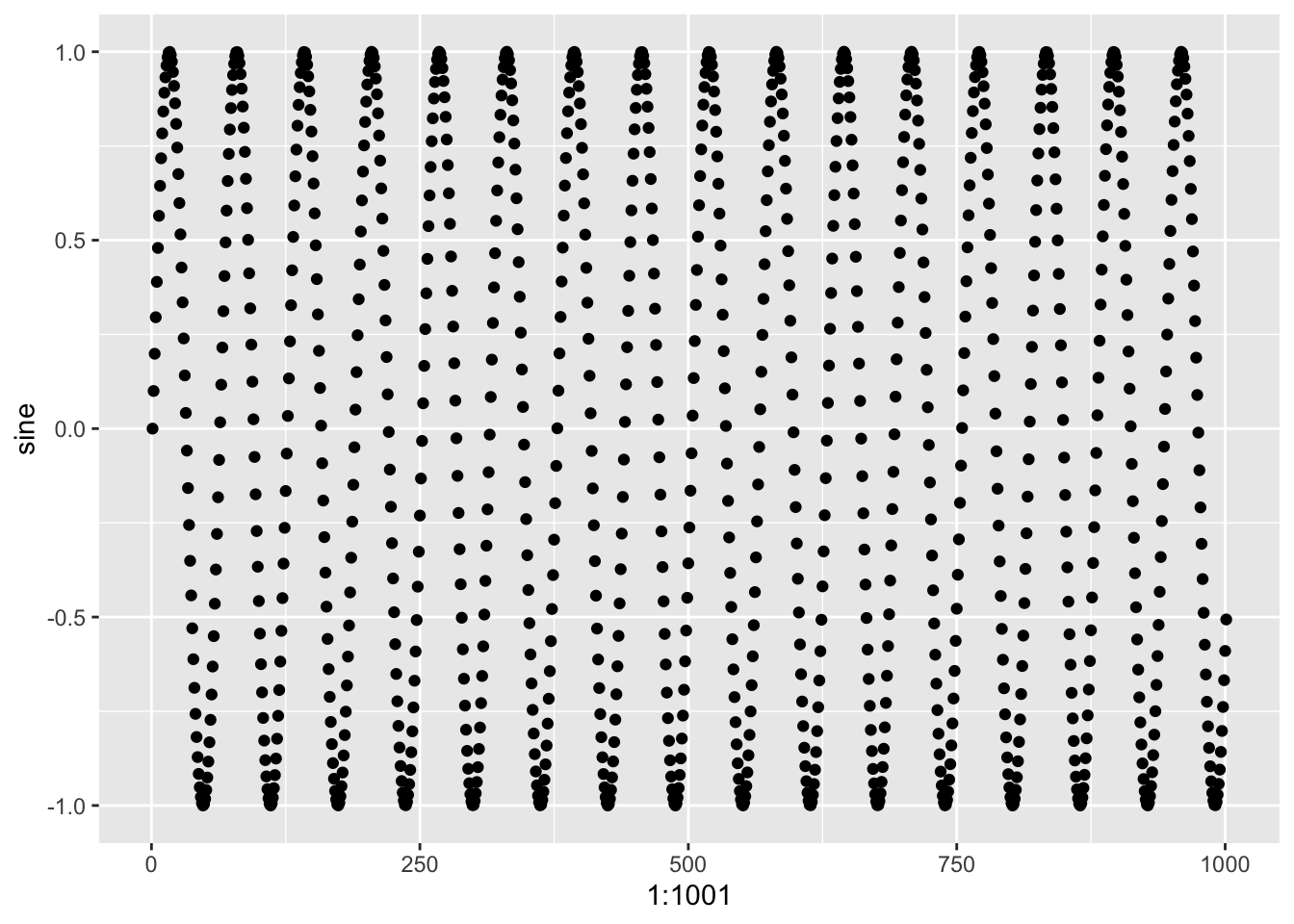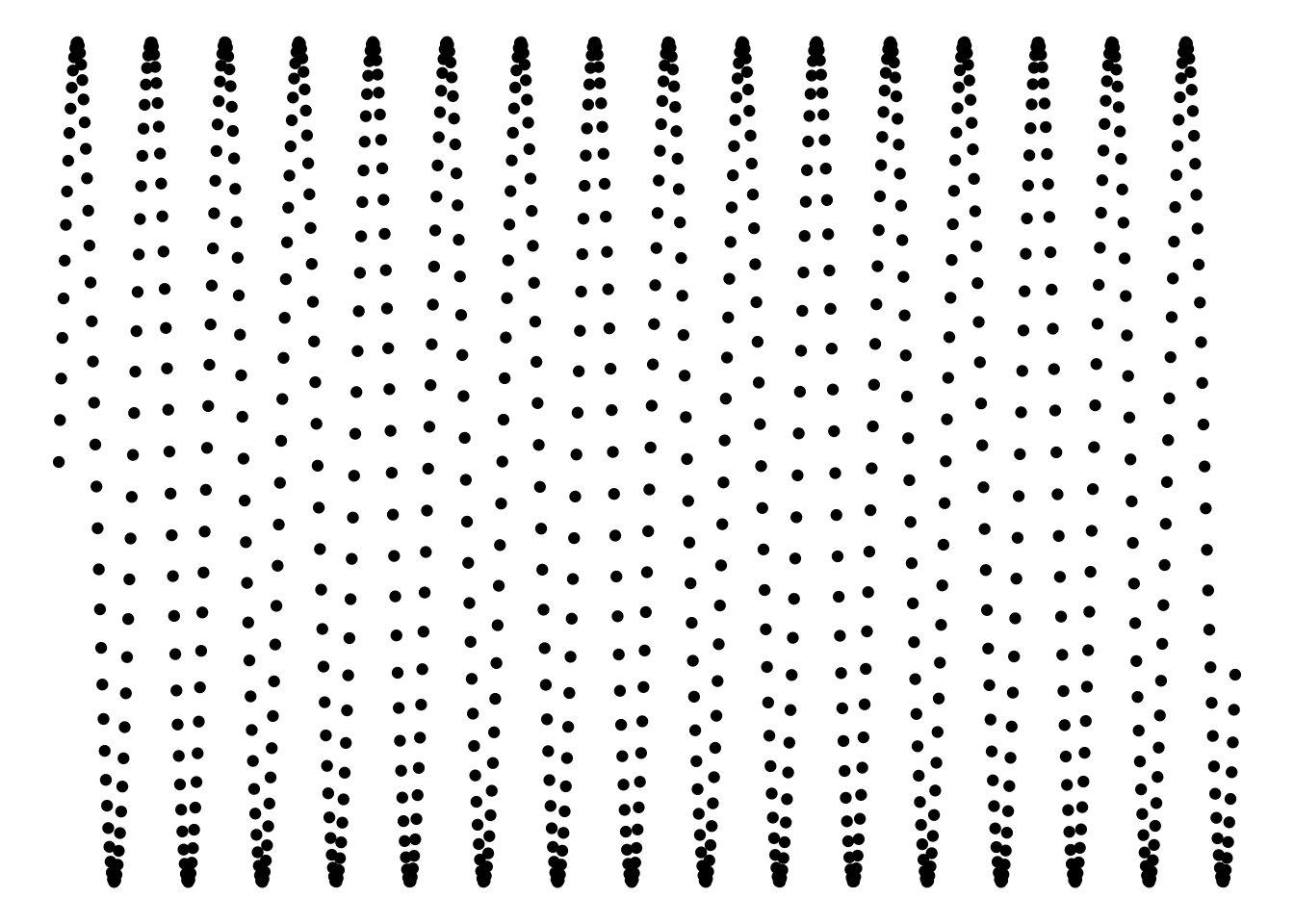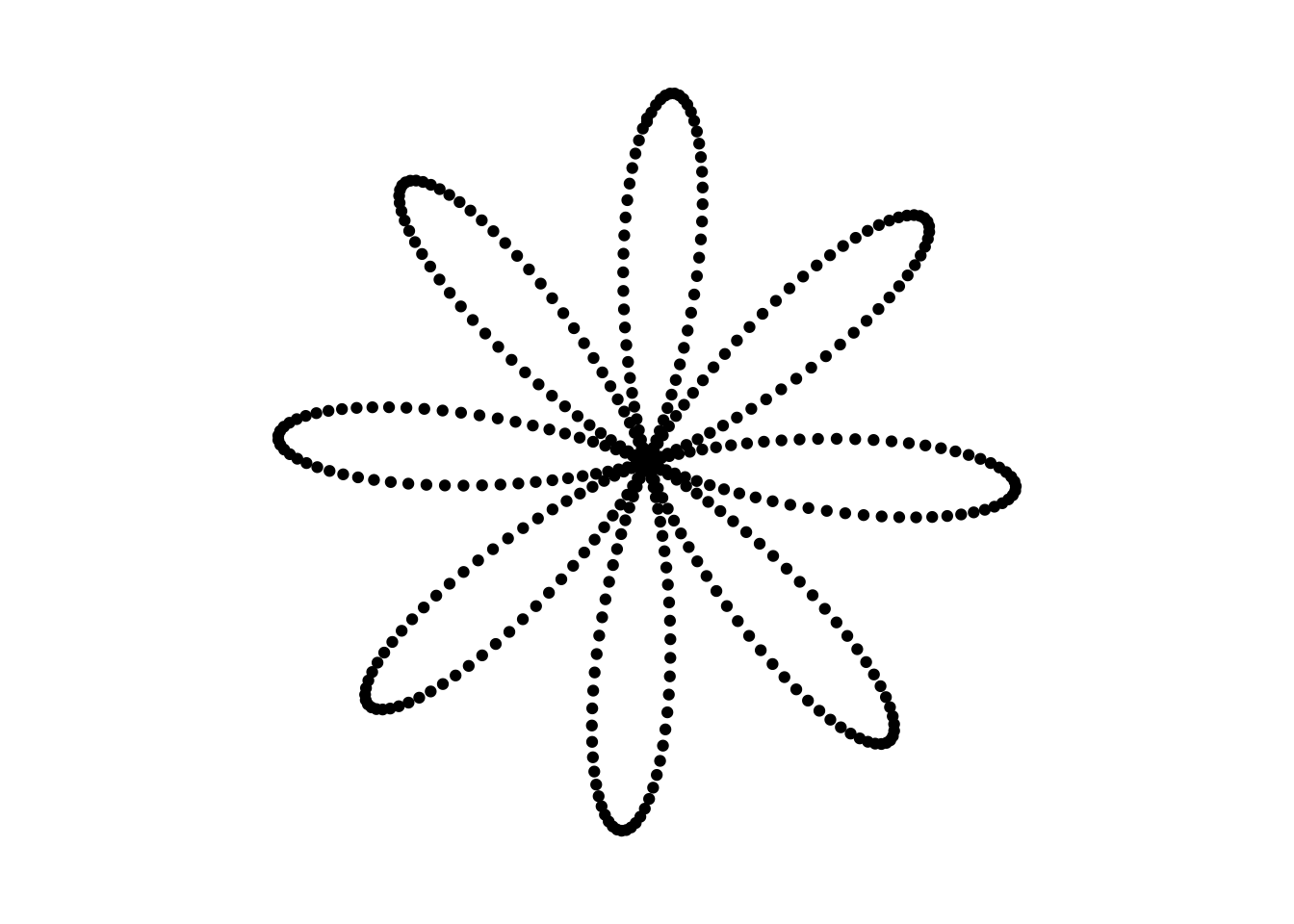# Solutions

As mentioned within the session setup, load the following packages using the `library()` function.

``````  library(tidyverse)
library(RColorBrewer)
library(ghibli)
library(palettetown)``````

Exercise 1: Using the data provided (`ex1.dat`), generated by the code below, plot the data onto a scatterplot using `ggplot()`. Plotting the variable `sine` from the data onto the x variable, and the index (`1:1001`) onto the y variable

Setup Code

``````# Generate the sequence 1 to 100, in steps of 0.1
ex1.dat <- as.data.frame(
seq(from = 0,
to = 100,
by = 0.1))

# Apply the sine function
ex1.dat <- sin(ex1.dat)

# Rename the columns
colnames(ex1.dat) <- "sine"``````

Solution

``````  ggplot(data = ex1.dat,
mapping = aes(x = 1:1001,
y = sine)) +
geom_point()``````Exercise 1, Bonus Question: Rather than using geom_point(), use geom_line() or another geom_ function to plot this same data in another way.

``````  ggplot(data = ex1.dat,
mapping = aes(x = 1:1001,
y = sine)) +
geom_line()``````Exercise 2: Add one of the two following themes to clean up your code!

``````  ggplot(data = ex1.dat,
mapping = aes(x = 1:1001,
y = sine)) +
geom_point() +
theme_void()``````Exercise 3/Bonus: Combined Mathematical Sequences, Using the same data as before (ex1.dat) transform the data and layer it onto the previous plots. Use the geom_line() function to more easily observe the impact of this transformation.

``````  ggplot() +
geom_line(data = (ex1.dat + 1),
mapping = aes(x = 1:1001, y = sine)) +
geom_line(data = (ex1.dat - 1),
mapping = aes(x = 1:1001, y = sine)) +

geom_line(data = (ex1.dat * 2),
mapping = aes(x = 1:1001, y = sine)) +

geom_line(data = (ex1.dat / 2),
mapping = aes(x = 1:1001, y = sine)) +

theme_void()``````Exercise 4: Changing Coordinate System

``````ex4.dat <- as.data.frame(seq(from = 1,
to = 51.3,
by = 0.1))
ex4.dat <- sin(ex4.dat)
colnames(ex4.dat) <- "sine"

ggplot(data = ex4.dat,
mapping = aes(x = 1:504,
y = sine)) +
geom_point() +
theme_void() +
coord_polar()``````Exercise 4, Bonus

``````ex4.dat <- as.data.frame(seq(from = 1,
to = 51.3,
by = 0.1))
colnames(ex4.dat) <- "seq"
ex4.dat\$sine <- sin(ex4.dat\$seq)
ex4.dat\$cos <- cos(ex4.dat\$seq)
ex4.dat\$tan <- tan(ex4.dat\$seq)

ggplot(data = ex4.dat) +
geom_point(mapping = aes(x = 1:504, y = sine)) +
geom_point(mapping = aes(x = 1:504, y = cos)) +
geom_point(mapping = aes(x = 1:504, y = tan)) +
theme_void() +
coord_polar() +
ylim(-1, 1)``````
``## Warning: Removed 251 rows containing missing values (geom_point).``Exercise 5: Layering Colours, using the code created in Exercise 4, replace `geom_point()` with `geom_polygon()` and apply a colour within using `fill =` or `colour =`.

``````ggplot(data = ex4.dat) +
geom_polygon(mapping = aes(x = 1:504, y = sine), fill = "blue") +
theme_void() +
coord_polar() +
ylim(-1, 1)``````Exercise 6: See inspiration for ideas about what you could do!